# UNIT 4 PDF FORMAT.pdf

29 de May de 2023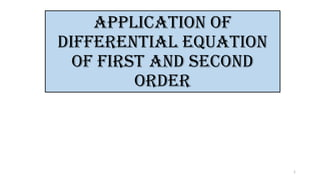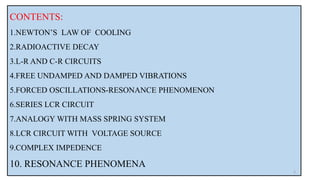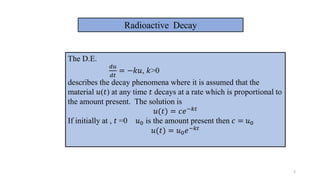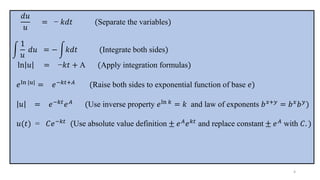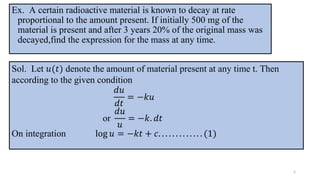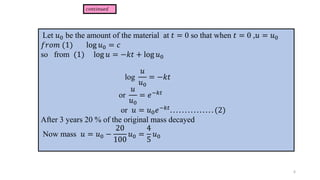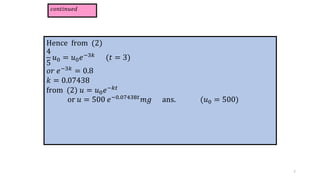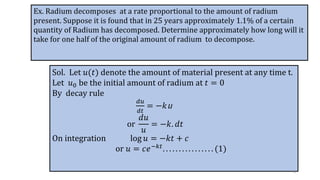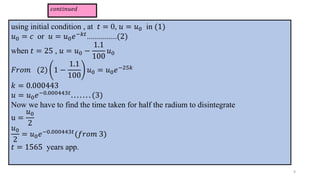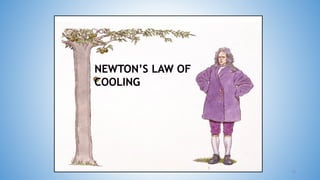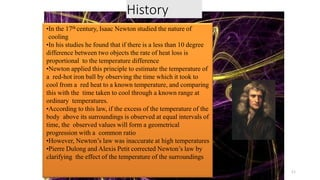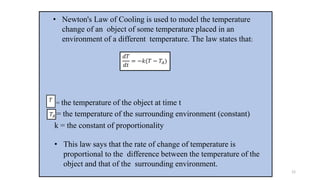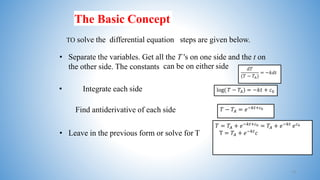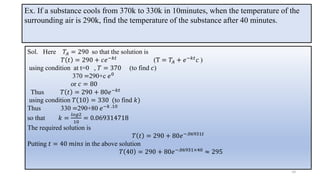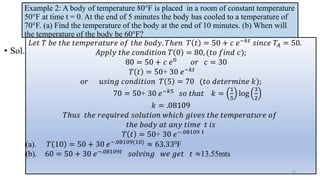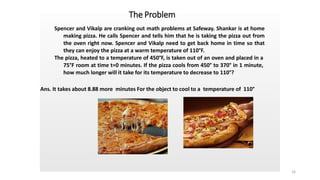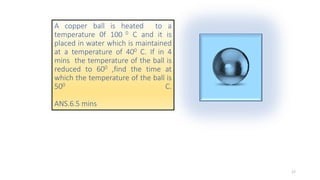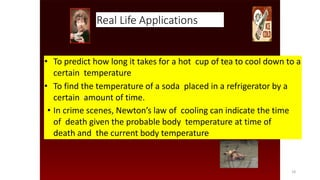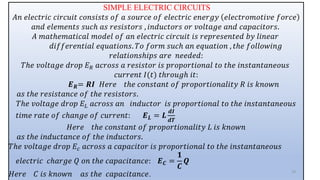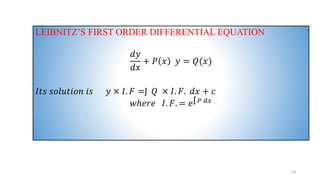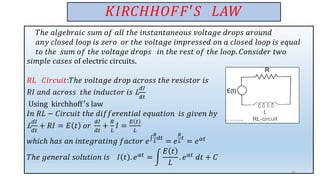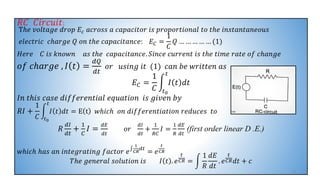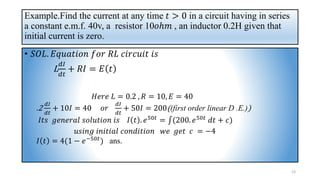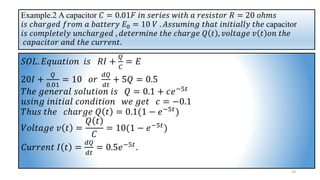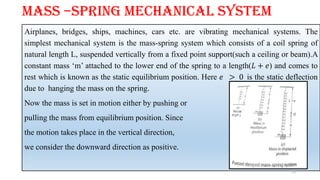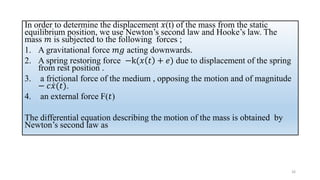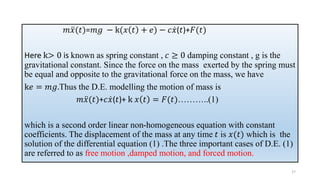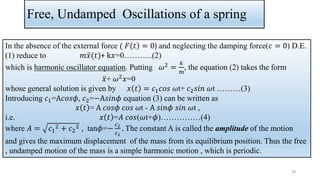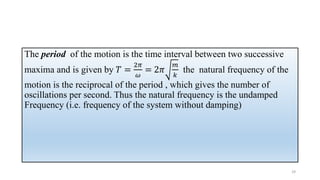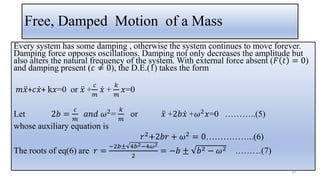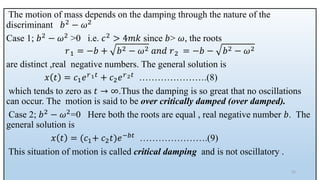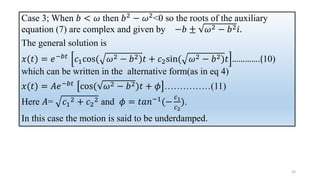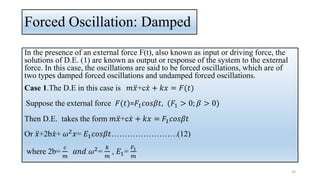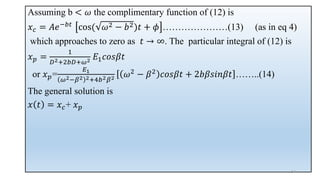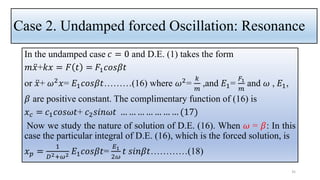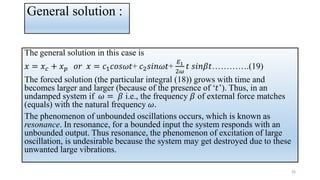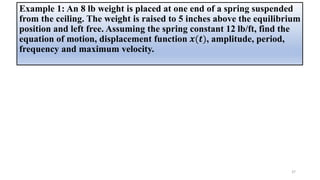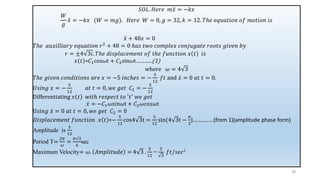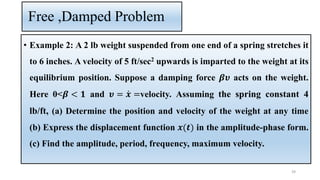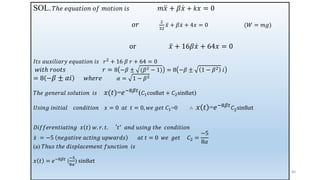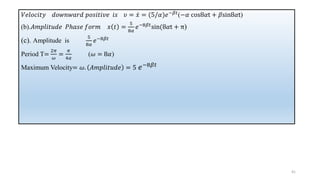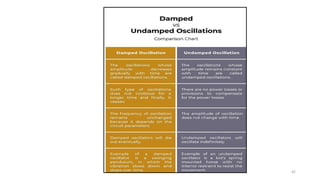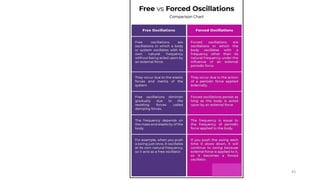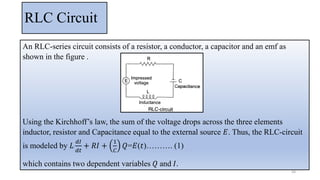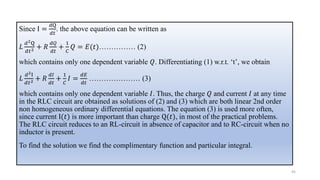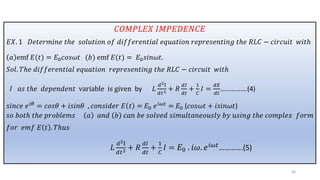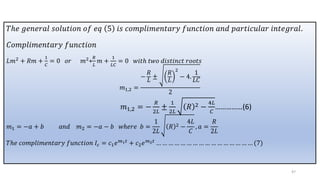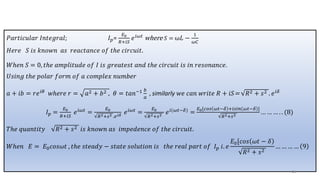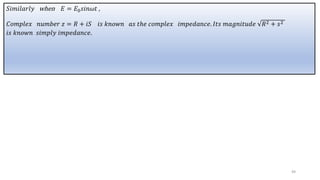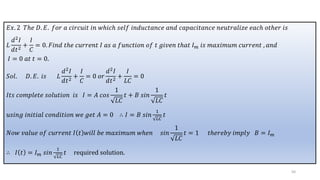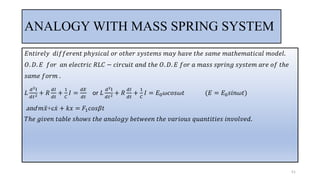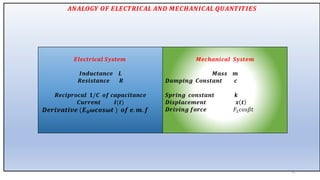1 de 53

### UNIT 4 PDF FORMAT.pdf

• 1. APPLICATION OF DIFFERENTIAL EQUATION OF First and SECOND ORDER 1
• 2. CONTENTS: 1.NEWTON’S LAW OF COOLING 2.RADIOACTIVE DECAY 3.L-R AND C-R CIRCUITS 4.FREE UNDAMPED AND DAMPED VIBRATIONS 5.FORCED OSCILLATIONS-RESONANCE PHENOMENON 6.SERIES LCR CIRCUIT 7.ANALOGY WITH MASS SPRING SYSTEM 8.LCR CIRCUIT WITH VOLTAGE SOURCE 9.COMPLEX IMPEDENCE 10. RESONANCE PHENOMENA 2
• 3. 3 Radioactive Decay The D.E. 𝑑𝑢 𝑑𝑡 = −𝑘𝑢, 𝑘>0 describes the decay phenomena where it is assumed that the material 𝑢(𝑡) at any time 𝑡 decays at a rate which is proportional to the amount present. The solution is 𝑢(𝑡) = 𝑐𝑒−𝑘𝑡 If initially at , 𝑡 =0 𝑢0 is the amount present then 𝑐 = 𝑢0 𝑢(𝑡) = 𝑢0𝑒−𝑘𝑡
• 4. 𝑑𝑢 𝑢 = − 𝑘𝑑𝑡 (Separate the variables) න 1 𝑢 𝑑𝑢 = − න𝑘𝑑𝑡 (Integrate both sides) ln 𝑢 = −𝑘𝑡 + A (Apply integration formulas) 𝑒ln |𝑢| = 𝑒−𝑘𝑡+𝐴 (Raise both sides to exponential function of base 𝑒) 𝑢 = 𝑒−𝑘𝑡 𝑒𝐴 (Use inverse property 𝑒ln 𝑘 = 𝑘 and law of exponents 𝑏𝑥+𝑦 = 𝑏𝑥 𝑏𝑦 ) 𝑢(𝑡) = 𝐶𝑒−𝑘𝑡 (Use absolute value definition ± 𝑒𝐴 𝑒𝑘𝑡 and replace constant ± 𝑒𝐴 with 𝐶. ) 4
• 5. Ex. A certain radioactive material is known to decay at rate proportional to the amount present. If initially 500 mg of the material is present and after 3 years 20% of the original mass was decayed,find the expression for the mass at any time. 5 Sol. Let 𝑢(𝑡) denote the amount of material present at any time t. Then according to the given condition 𝑑𝑢 𝑑𝑡 = −𝑘𝑢 or 𝑑𝑢 𝑢 = −𝑘. 𝑑𝑡 On integration log 𝑢 = −𝑘𝑡 + 𝑐. . . . . . . . . . . . . (1)
• 6. Let 𝑢0 be the amount of the material at 𝑡 = 0 so that when 𝑡 = 0 ,𝑢 = 𝑢0 𝑓𝑟𝑜𝑚 (1) log 𝑢0 = 𝑐 so from (1) log 𝑢 = −𝑘𝑡 + log 𝑢0 log 𝑢 𝑢0 = −𝑘𝑡 or 𝑢 𝑢0 = 𝑒−𝑘𝑡 or 𝑢 = 𝑢0𝑒−𝑘𝑡 . . . . . . . . . . . . . . . (2) After 3 years 20 % of the original mass decayed Now mass 𝑢 = 𝑢0 − 20 100 𝑢0 = 4 5 𝑢0 𝑐𝑜𝑛𝑡𝑖𝑛𝑢𝑒𝑑 6
• 7. 𝑐𝑜𝑛𝑡𝑖𝑛𝑢𝑒𝑑 Hence from (2) 4 5 𝑢0 = 𝑢0𝑒−3𝑘 (𝑡 = 3) 𝑜𝑟 𝑒−3𝑘 = 0.8 𝑘 = 0.07438 from (2) 𝑢 = 𝑢0𝑒−𝑘𝑡 or 𝑢 = 500 𝑒−0.07438𝑡𝑚𝑔 ans. (𝑢0 = 500) 7
• 8. Ex. Radium decomposes at a rate proportional to the amount of radium present. Suppose it is found that in 25 years approximately 1.1% of a certain quantity of Radium has decomposed. Determine approximately how long will it take for one half of the original amount of radium to decompose. Sol. Let 𝑢(𝑡) denote the amount of material present at any time t. Let 𝑢0 be the initial amount of radium at 𝑡 = 0 By decay rule 𝑑𝑢 𝑑𝑡 = −𝑘u or 𝑑𝑢 𝑢 = −𝑘. 𝑑𝑡 On integration log 𝑢 = −𝑘𝑡 + 𝑐 or 𝑢 = 𝑐𝑒−𝑘𝑡. . . . . . . . . . . . . . . . (1) 8
• 9. 𝑐𝑜𝑛𝑡𝑖𝑛𝑢𝑒𝑑 using initial condition , at 𝑡 = 0, 𝑢 = 𝑢0 in (1) 𝑢0 = 𝑐 or 𝑢 = 𝑢0𝑒−𝑘𝑡...............(2) when 𝑡 = 25 , 𝑢 = 𝑢0 − 1.1 100 𝑢0 𝐹𝑟𝑜𝑚 (2) 1 − 1.1 100 𝑢0 = 𝑢0𝑒−25𝑘 𝑘 = 0.000443 𝑢 = 𝑢0𝑒−0.000443𝑡. . . . . . . (3) Now we have to find the time taken for half the radium to disintegrate u = 𝑢0 2 𝑢0 2 = 𝑢0𝑒−0.000443𝑡(𝑓𝑟𝑜𝑚 3) 𝑡 = 1565 years app. 9
• 10. 1 10
• 11. History •In the 17th century, Isaac Newton studied the nature of cooling •In his studies he found that if there is a less than 10 degree difference between two objects the rate of heat loss is proportional to the temperature difference •Newton applied this principle to estimate the temperature of a red-hot iron ball by observing the time which it took to cool from a red heat to a known temperature, and comparing this with the time taken to cool through a known range at ordinary temperatures. •According to this law, if the excess of the temperature of the body above its surroundings is observed at equal intervals of time, the observed values will form a geometrical progression with a common ratio •However, Newton’s law was inaccurate at high temperatures •Pierre Dulong and Alexis Petit corrected Newton’s law by clarifying the effect of the temperature of the surroundings 11
• 12. • Newton's Law of Cooling is used to model the temperature change of an object of some temperature placed in an environment of a different temperature. The law states that: = the temperature of the object at time t = the temperature of the surrounding environment (constant) k = the constant of proportionality • This law says that the rate of change of temperature is proportional to the difference between the temperature of the object and that of the surrounding environment. 𝑑𝑇 𝑑𝑡 = −𝑘(𝑇 − 𝑇𝐴) 𝑇𝐴 𝑇 12
• 13. The Basic Concept TO solve the differential equation steps are given below. • Separate the variables. Get all the T 's on one side and the t on the other side. The constants can be on either side. • Integrate each side • Leave in the previous form or solve for T Find antiderivative of each side 𝑑𝑇 (𝑇 − 𝑇𝐴) = −𝑘𝑑𝑡 log( 𝑇 − 𝑇𝐴) = −𝑘𝑡 + 𝑐0 𝑇 − 𝑇𝐴 = 𝑒−𝑘𝑡+𝑐0 𝑇 = 𝑇𝐴 + 𝑒−𝑘𝑡+𝑐0 = 𝑇𝐴 + 𝑒−𝑘𝑡 𝑒𝑐0 T = 𝑇𝐴 + 𝑒−𝑘𝑡 𝑐 13
• 14. Ex. If a substance cools from 370k to 330k in 10minutes, when the temperature of the surrounding air is 290k, find the temperature of the substance after 40 minutes. Sol. Here 𝑇𝐴 = 290 so that the solution is 𝑇 𝑡 = 290 + 𝑐𝑒−𝑘𝑡 (T = 𝑇𝐴 + 𝑒−𝑘𝑡 𝑐 ) using condition at t=0 , 𝑇 = 370 (to find 𝑐) 370 =290+c 𝑒0 or 𝑐 = 80 Thus 𝑇 𝑡 = 290 + 80𝑒−𝑘𝑡 using condition 𝑇 10 = 330 (to find 𝑘) Thus 330 =290+80 𝑒−𝑘 .10 so that 𝑘 = 𝑙𝑜𝑔2 10 = 0.069314718 The required solution is 𝑇 𝑡 = 290 + 80𝑒−.06931𝑡 Putting 𝑡 = 40 𝑚𝑖𝑛𝑠 in the above solution 𝑇 40 = 290 + 80𝑒−.06931×40 ≈ 295 14
• 15. Example 2: A body of temperature 80°F is placed in a room of constant temperature 50°F at time t = 0. At the end of 5 minutes the body has cooled to a temperature of 70°F. (a) Find the temperature of the body at the end of 10 minutes. (b) When will the temperature of the body be 60°F? • Sol. 𝐿𝑒𝑡 𝑇 𝑏𝑒 𝑡ℎ𝑒 𝑡𝑒𝑚𝑝𝑒𝑟𝑎𝑡𝑢𝑟𝑒 𝑜𝑓 𝑡ℎ𝑒 𝑏𝑜𝑑𝑦. 𝑇ℎ𝑒𝑛 𝑇 𝑡 = 50 + 𝑐 𝑒−𝑘𝑡 𝑠𝑖𝑛𝑐𝑒 𝑇𝐴 = 50. 𝐴𝑝𝑝𝑙𝑦 𝑡ℎ𝑒 𝑐𝑜𝑛𝑑𝑖𝑡𝑖𝑜𝑛 𝑇 0 = 80, (𝑡𝑜 𝑓𝑖𝑛𝑑 𝑐); 80 = 50 + 𝑐 𝑒0 𝑜𝑟 𝑐 = 30 𝑇 𝑡 = 50+ 30 𝑒−𝑘𝑡 o𝑟 𝑢𝑠𝑖𝑛𝑔 𝑐𝑜𝑛𝑑𝑖𝑡𝑖𝑜𝑛 𝑇 5 = 70 (𝑡𝑜 𝑑𝑒𝑡𝑒𝑟𝑚𝑖𝑛𝑒 𝑘); 70 = 50+ 30 𝑒−𝑘5 𝑠𝑜 𝑡ℎ𝑎𝑡 𝑘 = 1 5 log 3 2 𝑘 = .08109 𝑇ℎ𝑢𝑠 𝑡ℎ𝑒 𝑟𝑒𝑞𝑢𝑖𝑟𝑒𝑑 𝑠𝑜𝑙𝑢𝑡𝑖𝑜𝑛 𝑤ℎ𝑖𝑐ℎ 𝑔𝑖𝑣𝑒𝑠 𝑡ℎ𝑒 𝑡𝑒𝑚𝑝𝑒𝑟𝑎𝑡𝑢𝑟𝑒 𝑜𝑓 𝑡ℎ𝑒 𝑏𝑜𝑑𝑦 𝑎𝑡 𝑎𝑛𝑦 𝑡𝑖𝑚𝑒 𝑡 𝑖𝑠 𝑇 𝑡 = 50+ 30 𝑒−.08109 𝑡 (a). 𝑇 10 = 50 + 30 𝑒−.08109(10) ≈ 63.330F (b). 60 = 50 + 30 𝑒−.08109𝑡 𝑠𝑜𝑙𝑣𝑖𝑛𝑔 𝑤𝑒 𝑔𝑒𝑡 𝑡 ≈13.55mts 15
• 16. Ans. It takes about 8.88 more minutes For the object to cool to a temperature of 110° 110° For the object to cool to a temperature of 110° The Problem Spencer and Vikalp are cranking out math problems at Safeway. Shankar is at home making pizza. He calls Spencer and tells him that he is taking the pizza out from the oven right now. Spencer and Vikalp need to get back home in time so that they can enjoy the pizza at a warm temperature of 110°F. The pizza, heated to a temperature of 450°F, is taken out of an oven and placed in a 75°F room at time t=0 minutes. If the pizza cools from 450° to 370° in 1 minute, how much longer will it take for its temperature to decrease to 110°? 16
• 17. A copper ball is heated to a temperature 0f 100 0 C and it is placed in water which is maintained at a temperature of 400 C. If in 4 mins the temperature of the ball is reduced to 600 ,find the time at which the temperature of the ball is 500 C. ANS.6.5 mins 17
• 18. Real Life Applications • To predict how long it takes for a hot cup of tea to cool down to a certain temperature • To find the temperature of a soda placed in a refrigerator by a certain amount of time. • In crime scenes, Newton’s law of cooling can indicate the time of death given the probable body temperature at time of death and the current body temperature • 18
• 19. SIMPLE ELECTRIC CIRCUITS 𝐴𝑛 𝑒𝑙𝑒𝑐𝑡𝑟𝑖𝑐 𝑐𝑖𝑟𝑐𝑢𝑖𝑡 𝑐𝑜𝑛𝑠𝑖𝑠𝑡𝑠 𝑜𝑓 𝑎 𝑠𝑜𝑢𝑟𝑐𝑒 𝑜𝑓 𝑒𝑙𝑒𝑐𝑡𝑟𝑖𝑐 𝑒𝑛𝑒𝑟𝑔𝑦 𝑒𝑙𝑒𝑐𝑡𝑟𝑜𝑚𝑜𝑡𝑖𝑣𝑒 𝑓𝑜𝑟𝑐𝑒 𝑎𝑛𝑑 𝑒𝑙𝑒𝑚𝑒𝑛𝑡𝑠 𝑠𝑢𝑐ℎ 𝑎𝑠 𝑟𝑒𝑠𝑖𝑠𝑡𝑜𝑟𝑠 , 𝑖𝑛𝑑𝑢𝑐𝑡𝑜𝑟𝑠 𝑜𝑟 𝑣𝑜𝑙𝑡𝑎𝑔𝑒 𝑎𝑛𝑑 𝑐𝑎𝑝𝑎𝑐𝑖𝑡𝑜𝑟𝑠. 𝐴 𝑚𝑎𝑡ℎ𝑒𝑚𝑎𝑡𝑖𝑐𝑎𝑙 𝑚𝑜𝑑𝑒𝑙 𝑜𝑓 𝑎𝑛 𝑒𝑙𝑒𝑐𝑡𝑟𝑖𝑐 𝑐𝑖𝑟𝑐𝑢𝑖𝑡 𝑖𝑠 𝑟𝑒𝑝𝑟𝑒𝑠𝑒𝑛𝑡𝑒𝑑 𝑏𝑦 𝑙𝑖𝑛𝑒𝑎𝑟 𝑑𝑖𝑓𝑓𝑒𝑟𝑒𝑛𝑡𝑖𝑎𝑙 𝑒𝑞𝑢𝑎𝑡𝑖𝑜𝑛𝑠. 𝑇𝑜 𝑓𝑜𝑟𝑚 𝑠𝑢𝑐ℎ 𝑎𝑛 𝑒𝑞𝑢𝑎𝑡𝑖𝑜𝑛 , 𝑡ℎ𝑒 𝑓𝑜𝑙𝑙𝑜𝑤𝑖𝑛𝑔 𝑟𝑒𝑙𝑎𝑡𝑖𝑜𝑛𝑠ℎ𝑖𝑝𝑠 𝑎𝑟𝑒 𝑛𝑒𝑒𝑑𝑒𝑑: 𝑇ℎ𝑒 𝑣𝑜𝑙𝑡𝑎𝑔𝑒 𝑑𝑟𝑜𝑝 𝐸𝑅 𝑎𝑐𝑟𝑜𝑠𝑠 𝑎 𝑟𝑒𝑠𝑖𝑠𝑡𝑜𝑟 𝑖𝑠 𝑝𝑟𝑜𝑝𝑜𝑟𝑡𝑖𝑜𝑛𝑎𝑙 𝑡𝑜 𝑡ℎ𝑒 𝑖𝑛𝑠𝑡𝑎𝑛𝑡𝑎𝑛𝑒𝑜𝑢𝑠 𝑐𝑢𝑟𝑟𝑒𝑛𝑡 𝐼(𝑡) 𝑡ℎ𝑟𝑜𝑢𝑔ℎ 𝑖𝑡: 𝑬𝑹= 𝑹𝑰 𝐻𝑒𝑟𝑒 𝑡ℎ𝑒 𝑐𝑜𝑛𝑠𝑡𝑎𝑛𝑡 𝑜𝑓 𝑝𝑟𝑜𝑝𝑜𝑟𝑡𝑖𝑜𝑛𝑎𝑙𝑖𝑡𝑦 𝑅 𝑖𝑠 𝑘𝑛𝑜𝑤𝑛 𝑎𝑠 𝑡ℎ𝑒 𝑟𝑒𝑠𝑖𝑠𝑡𝑎𝑛𝑐𝑒 𝑜𝑓 𝑡ℎ𝑒 𝑟𝑒𝑠𝑖𝑠𝑡𝑜𝑟𝑠. 𝑇ℎ𝑒 𝑣𝑜𝑙𝑡𝑎𝑔𝑒 𝑑𝑟𝑜𝑝 𝐸𝐿 𝑎𝑐𝑟𝑜𝑠𝑠 𝑎𝑛 𝑖𝑛𝑑𝑢𝑐𝑡𝑜𝑟 𝑖𝑠 𝑝𝑟𝑜𝑝𝑜𝑟𝑡𝑖𝑜𝑛𝑎𝑙 𝑡𝑜 𝑡ℎ𝑒 𝑖𝑛𝑠𝑡𝑎𝑛𝑡𝑎𝑛𝑒𝑜𝑢𝑠 𝑡𝑖𝑚𝑒 𝑟𝑎𝑡𝑒 𝑜𝑓 𝑐ℎ𝑎𝑛𝑔𝑒 𝑜𝑓 𝑐𝑢𝑟𝑟𝑒𝑛𝑡: 𝑬𝑳 = 𝑳 𝒅𝑰 𝒅𝑻 𝐻𝑒𝑟𝑒 𝑡ℎ𝑒 𝑐𝑜𝑛𝑠𝑡𝑎𝑛𝑡 𝑜𝑓 𝑝𝑟𝑜𝑝𝑜𝑟𝑡𝑖𝑜𝑛𝑎𝑙𝑖𝑡𝑦 𝐿 𝑖𝑠 𝑘𝑛𝑜𝑤𝑛 𝑎𝑠 𝑡ℎ𝑒 𝑖𝑛𝑑𝑢𝑐𝑡𝑎𝑛𝑐𝑒 𝑜𝑓 𝑡ℎ𝑒 𝑖𝑛𝑑𝑢𝑐𝑡𝑜𝑟𝑠. 𝑇ℎ𝑒 𝑣𝑜𝑙𝑡𝑎𝑔𝑒 𝑑𝑟𝑜𝑝 𝐸𝑐 𝑎𝑐𝑟𝑜𝑠𝑠 𝑎 𝑐𝑎𝑝𝑎𝑐𝑖𝑡𝑜𝑟 𝑖𝑠 𝑝𝑟𝑜𝑝𝑜𝑟𝑡𝑖𝑜𝑛𝑎𝑙 𝑡𝑜 𝑡ℎ𝑒 𝑖𝑛𝑠𝑡𝑎𝑛𝑡𝑎𝑛𝑒𝑜𝑢𝑠 𝑒𝑙𝑒𝑐𝑡𝑟𝑖𝑐 𝑐ℎ𝑎𝑟𝑔𝑒 𝑄 𝑜𝑛 𝑡ℎ𝑒 𝑐𝑎𝑝𝑎𝑐𝑖𝑡𝑎𝑛𝑐𝑒: 𝑬𝑪 = 𝟏 𝑪 𝑸 𝐻𝑒𝑟𝑒 𝐶 𝑖𝑠 𝑘𝑛𝑜𝑤𝑛 𝑎𝑠 𝑡ℎ𝑒 𝑐𝑎𝑝𝑎𝑐𝑖𝑡𝑎𝑛𝑐𝑒. 19
• 20. LEIBNITZ’S FIRST ORDER DIFFERENTIAL EQUATION 𝑑𝑦 𝑑𝑥 + 𝑃 𝑥 𝑦 = 𝑄(𝑥) 𝐼𝑡𝑠 𝑠𝑜𝑙𝑢𝑡𝑖𝑜𝑛 𝑖𝑠 𝑦 × 𝐼. 𝐹 =ʃ 𝑄 × 𝐼. 𝐹. 𝑑𝑥 + 𝑐 𝑤ℎ𝑒𝑟𝑒 𝐼. 𝐹. = 𝑒ʃ 𝑃 𝑑𝑥 20
• 21. 𝐾𝐼𝑅𝐶𝐻𝐻𝑂𝐹𝐹′𝑆 𝐿𝐴𝑊 𝑇ℎ𝑒 𝑎𝑙𝑔𝑒𝑏𝑟𝑎𝑖𝑐 𝑠𝑢𝑚 𝑜𝑓 𝑎𝑙𝑙 𝑡ℎ𝑒 𝑖𝑛𝑠𝑡𝑎𝑛𝑡𝑎𝑛𝑒𝑜𝑢𝑠 𝑣𝑜𝑙𝑡𝑎𝑔𝑒 𝑑𝑟𝑜𝑝𝑠 𝑎𝑟𝑜𝑢𝑛𝑑 𝑎𝑛𝑦 𝑐𝑙𝑜𝑠𝑒𝑑 𝑙𝑜𝑜𝑝 𝑖𝑠 𝑧𝑒𝑟𝑜 𝑜𝑟 𝑡ℎ𝑒 𝑣𝑜𝑙𝑡𝑎𝑔𝑒 𝑖𝑚𝑝𝑟𝑒𝑠𝑠𝑒𝑑 𝑜𝑛 𝑎 𝑐𝑙𝑜𝑠𝑒𝑑 𝑙𝑜𝑜𝑝 𝑖𝑠 𝑒𝑞𝑢𝑎𝑙 𝑡𝑜 𝑡ℎ𝑒 𝑠𝑢𝑚 𝑜𝑓 𝑡ℎ𝑒 𝑣𝑜𝑙𝑡𝑎𝑔𝑒 𝑑𝑟𝑜𝑝𝑠 𝑖𝑛 𝑡ℎ𝑒 𝑟𝑒𝑠𝑡 𝑜𝑓 𝑡ℎ𝑒 𝑙𝑜𝑜𝑝. 𝐶𝑜𝑛𝑠𝑖𝑑𝑒𝑟 𝑡𝑤𝑜 𝑠𝑖𝑚𝑝𝑙𝑒 𝑐𝑎𝑠𝑒𝑠 of electric circuits. 𝑅𝐿 𝐶𝑖𝑟𝑐𝑢𝑖𝑡:𝑇ℎ𝑒 𝑣𝑜𝑙𝑡𝑎𝑔𝑒 𝑑𝑟𝑜𝑝 𝑎𝑐𝑟𝑜𝑠𝑠 𝑡ℎ𝑒 𝑟𝑒𝑠𝑖𝑠𝑡𝑜𝑟 𝑖𝑠 𝑅𝐼 𝑎𝑛𝑑 𝑎𝑐𝑟𝑜𝑠𝑠 𝑡ℎ𝑒 𝑖𝑛𝑑𝑢𝑐𝑡𝑜𝑟 𝑖𝑠 L 𝑑𝐼 𝑑𝑡 Using kirchhoff′s law 𝐼𝑛 𝑅𝐿 − 𝐶𝑖𝑟𝑐𝑢𝑖𝑡 𝑡ℎ𝑒 𝑑𝑖𝑓𝑓𝑒𝑟𝑒𝑛𝑡𝑖𝑎𝑙 𝑒𝑞𝑢𝑎𝑡𝑖𝑜𝑛 𝑖𝑠 𝑔𝑖𝑣𝑒𝑛 𝑏𝑦 L 𝑑𝐼 𝑑𝑡 + 𝑅𝐼 = 𝐸 𝑡 𝑜𝑟 𝑑𝐼 𝑑𝑡 + 𝑅 𝐿 𝐼 = 𝐸 𝑡 𝐿 𝑤ℎ𝑖𝑐ℎ ℎ𝑎𝑠 𝑎𝑛 𝑖𝑛𝑡𝑒𝑔𝑟𝑎𝑡𝑖𝑛𝑔 𝑓𝑎𝑐𝑡𝑜𝑟 𝑒‫׬‬ 𝑅 𝐿 𝑑𝑡 = 𝑒 𝑅 𝐿 𝑡 = 𝑒𝛼𝑡 𝑇ℎ𝑒 𝑔𝑒𝑛𝑒𝑟𝑎𝑙 𝑠𝑜𝑙𝑢𝑡𝑖𝑜𝑛 𝑖𝑠 𝐼 𝑡 . 𝑒𝛼𝑡 = න 𝐸 𝑡 𝐿 . 𝑒𝛼𝑡 𝑑𝑡 + 𝐶 21
• 22. 𝑅𝐶 𝐶𝑖𝑟𝑐𝑢𝑖𝑡: Tℎ𝑒 𝑣𝑜𝑙𝑡𝑎𝑔𝑒 𝑑𝑟𝑜𝑝 𝐸𝑐 𝑎𝑐𝑟𝑜𝑠𝑠 𝑎 𝑐𝑎𝑝𝑎𝑐𝑖𝑡𝑜𝑟 𝑖𝑠 𝑝𝑟𝑜𝑝𝑜𝑟𝑡𝑖𝑜𝑛𝑎𝑙 𝑡𝑜 𝑡ℎ𝑒 𝑖𝑛𝑠𝑡𝑎𝑛𝑡𝑎𝑛𝑒𝑜𝑢𝑠 𝑒𝑙𝑒𝑐𝑡𝑟𝑖𝑐 𝑐ℎ𝑎𝑟𝑔𝑒 𝑄 𝑜𝑛 𝑡ℎ𝑒 𝑐𝑎𝑝𝑎𝑐𝑖𝑡𝑎𝑛𝑐𝑒: 𝐸𝐶 = 1 𝐶 𝑄 … … … … … (1) 𝐻𝑒𝑟𝑒 𝐶 𝑖𝑠 𝑘𝑛𝑜𝑤𝑛 𝑎𝑠 𝑡ℎ𝑒 𝑐𝑎𝑝𝑎𝑐𝑖𝑡𝑎𝑛𝑐𝑒. 𝑆𝑖𝑛𝑐𝑒 𝑐𝑢𝑟𝑟𝑒𝑛𝑡 𝑖𝑠 𝑡ℎ𝑒 𝑡𝑖𝑚𝑒 𝑟𝑎𝑡𝑒 𝑜𝑓 𝑐ℎ𝑎𝑛𝑔𝑒 𝑜𝑓 𝑐ℎ𝑎𝑟𝑔𝑒 , 𝐼 𝑡 = 𝑑𝑄 𝑑𝑡 𝑜𝑟 𝑢𝑠𝑖𝑛𝑔 𝑖𝑡 (1) 𝑐𝑎𝑛 𝑏𝑒 𝑤𝑟𝑖𝑡𝑡𝑒𝑛 𝑎𝑠 𝐸𝐶 = 1 𝐶 න 𝑡0 𝑡 𝐼 𝑡 𝑑𝑡 𝐼𝑛 𝑡ℎ𝑖𝑠 𝑐𝑎𝑠𝑒 𝑑𝑖𝑓𝑓𝑒𝑟𝑒𝑛𝑡𝑖𝑎𝑙 𝑒𝑞𝑢𝑎𝑡𝑖𝑜𝑛 𝑖𝑠 𝑔𝑖𝑣𝑒𝑛 𝑏𝑦 𝑅𝐼 + 1 𝐶 න 𝑡0 𝑡 𝐼 𝑡 𝑑𝑡 = E t 𝑤ℎ𝑖𝑐ℎ 𝑜𝑛 𝑑𝑖𝑓𝑓𝑒𝑟𝑒𝑛𝑡𝑖𝑎𝑡𝑖𝑜𝑛 𝑟𝑒𝑑𝑢𝑐𝑒𝑠 𝑡𝑜 𝑅 𝑑𝐼 𝑑𝑡 + 1 𝐶 𝐼 = 𝑑𝐸 𝑑𝑡 𝑜𝑟 𝑑𝐼 𝑑𝑡 + 1 𝑅𝐶 𝐼 = 1 𝑅 𝑑𝐸 𝑑𝑡 (first order linear D .E.) 𝑤ℎ𝑖𝑐ℎ ℎ𝑎𝑠 𝑎𝑛 𝑖𝑛𝑡𝑒𝑔𝑟𝑎𝑡𝑖𝑛𝑔 𝑓𝑎𝑐𝑡𝑜𝑟 𝑒‫׬‬ 1 𝐶𝑅 𝑑𝑡 = 𝑒 𝑡 𝐶𝑅 𝑇ℎ𝑒 𝑔𝑒𝑛𝑒𝑟𝑎𝑙 𝑠𝑜𝑙𝑢𝑡𝑖𝑜𝑛 𝑖𝑠 𝐼 𝑡 . 𝑒 𝑡 𝐶𝑅 = න 1 𝑅 𝑑𝐸 𝑑𝑡 . 𝑒 𝑡 𝐶𝑅𝑑𝑡 + 𝑐 22
• 23. Example.Find the current at any time 𝑡 > 0 in a circuit having in series a constant e.m.f. 40v, a resistor 10𝑜ℎ𝑚 , an inductor 0.2H given that initial current is zero. • 𝑆𝑂𝐿. 𝐸𝑞𝑢𝑎𝑡𝑖𝑜𝑛 𝑓𝑜𝑟 𝑅𝐿 𝑐𝑖𝑟𝑐𝑢𝑖𝑡 𝑖𝑠 L 𝑑𝐼 𝑑𝑡 + 𝑅𝐼 = 𝐸 𝑡 𝐻𝑒𝑟𝑒 𝐿 = 0.2 , 𝑅 = 10, 𝐸 = 40 .2 𝑑𝐼 𝑑𝑡 + 10𝐼 = 40 𝑜𝑟 𝑑𝐼 𝑑𝑡 + 50𝐼 = 200((first order linear D .E.)) 𝐼𝑡𝑠 𝑔𝑒𝑛𝑒𝑟𝑎𝑙 𝑠𝑜𝑙𝑢𝑡𝑖𝑜𝑛 𝑖𝑠 𝐼 𝑡 . 𝑒50𝑡 = ‫׬‬(200. 𝑒50𝑡 𝑑𝑡 + 𝑐) 𝑢𝑠𝑖𝑛𝑔 𝑖𝑛𝑖𝑡𝑖𝑎𝑙 𝑐𝑜𝑛𝑑𝑖𝑡𝑖𝑜𝑛 𝑤𝑒 𝑔𝑒𝑡 𝑐 = −4 𝐼 𝑡 = 4(1 − 𝑒−50𝑡) ans. 23
• 24. Example.2 A capacitor 𝐶 = 0.01𝐹 𝑖𝑛 𝑠𝑒𝑟𝑖𝑒𝑠 𝑤𝑖𝑡ℎ 𝑎 𝑟𝑒𝑠𝑖𝑠𝑡𝑜𝑟 𝑅 = 20 𝑜ℎ𝑚𝑠 𝑖𝑠 𝑐ℎ𝑎𝑟𝑔𝑒𝑑 𝑓𝑟𝑜𝑚 𝑎 𝑏𝑎𝑡𝑡𝑒𝑟𝑦 𝐸0 = 10 𝑉 . 𝐴𝑠𝑠𝑢𝑚𝑖𝑛𝑔 𝑡ℎ𝑎𝑡 𝑖𝑛𝑖𝑡𝑖𝑎𝑙𝑙𝑦 𝑡ℎ𝑒 capacitor 𝑖𝑠 𝑐𝑜𝑚𝑝𝑙𝑒𝑡𝑒𝑙𝑦 𝑢𝑛𝑐ℎ𝑎𝑟𝑔𝑒𝑑 , 𝑑𝑒𝑡𝑒𝑟𝑚𝑖𝑛𝑒 𝑡ℎ𝑒 𝑐ℎ𝑎𝑟𝑔𝑒 𝑄 𝑡 , 𝑣𝑜𝑙𝑡𝑎𝑔𝑒 𝑣 𝑡 𝑜𝑛 𝑡ℎ𝑒 𝑐𝑎𝑝𝑎𝑐𝑖𝑡𝑜𝑟 𝑎𝑛𝑑 𝑡ℎ𝑒 𝑐𝑢𝑟𝑟𝑒𝑛𝑡. 𝑆𝑂𝐿. 𝐸𝑞𝑢𝑎𝑡𝑖𝑜𝑛 𝑖𝑠 𝑅𝐼 + 𝑄 𝐶 = 𝐸 20𝐼 + 𝑄 0.01 = 10 𝑜𝑟 𝑑𝑄 𝑑𝑡 + 5𝑄 = 0.5 𝑇ℎ𝑒 𝑔𝑒𝑛𝑒𝑟𝑎𝑙 𝑠𝑜𝑙𝑢𝑡𝑖𝑜𝑛 𝑖𝑠 𝑄 = 0.1 + 𝑐𝑒−5𝑡 𝑢𝑠𝑖𝑛𝑔 𝑖𝑛𝑖𝑡𝑖𝑎𝑙 𝑐𝑜𝑛𝑑𝑖𝑡𝑖𝑜𝑛 𝑤𝑒 𝑔𝑒𝑡 𝑐 = −0.1 𝑇ℎ𝑢𝑠 𝑡ℎ𝑒 𝑐ℎ𝑎𝑟𝑔𝑒 𝑄 𝑡 = 0.1(1 − 𝑒−5𝑡) 𝑉𝑜𝑙𝑡𝑎𝑔𝑒 𝑣 𝑡 = 𝑄 𝑡 𝐶 = 10(1 − 𝑒−5𝑡) 𝐶𝑢𝑟𝑟𝑒𝑛𝑡 𝐼 𝑡 = 𝑑𝑄 𝑑𝑡 = 0.5𝑒−5𝑡 . 24
• 25. MASS –SPRING MECHANICAL SYSTEM Airplanes, bridges, ships, machines, cars etc. are vibrating mechanical systems. The simplest mechanical system is the mass-spring system which consists of a coil spring of natural length L, suspended vertically from a fixed point support(such a ceiling or beam).A constant mass ‘m’ attached to the lower end of the spring to a length(𝐿 + 𝑒) and comes to rest which is known as the static equilibrium position. Here 𝑒 > 0 is the static deflection due to hanging the mass on the spring. Now the mass is set in motion either by pushing or pulling the mass from equilibrium position. Since the motion takes place in the vertical direction, we consider the downward direction as positive. 25
• 26. In order to determine the displacement 𝑥(t) of the mass from the static equilibrium position, we use Newton’s second law and Hooke’s law. The mass 𝑚 is subjected to the following forces ; 1. A gravitational force 𝑚𝑔 acting downwards. 2. A spring restoring force −k(𝑥 𝑡 + 𝑒) due to displacement of the spring from rest position . 3. a frictional force of the medium , opposing the motion and of magnitude − 𝑐 ሶ 𝑥(𝑡). 4. an external force F(𝑡) The differential equation describing the motion of the mass is obtained by Newton’s second law as 26
• 27. 𝑚 ሷ 𝑥(𝑡)=𝑚𝑔 − k(𝑥 𝑡 + 𝑒) − 𝑐 ሶ 𝑥(𝑡)+𝐹(𝑡) Here k> 0 is known as spring constant , 𝑐 ≥ 0 damping constant , g is the gravitational constant. Since the force on the mass exerted by the spring must be equal and opposite to the gravitational force on the mass, we have k𝑒 = 𝑚𝑔.Thus the D.E. modelling the motion of mass is 𝑚 ሷ 𝑥(𝑡)+𝑐 ሶ 𝑥(𝑡)+ k 𝑥 𝑡 = 𝐹(𝑡)………..(1) which is a second order linear non-homogeneous equation with constant coefficients. The displacement of the mass at any time 𝑡 is 𝑥(𝑡) which is the solution of the differential equation (1) .The three important cases of D.E. (1) are referred to as free motion ,damped motion, and forced motion. 27
• 28. Free, Undamped Oscillations of a spring In the absence of the external force ( 𝐹 𝑡 = 0) and neglecting the damping force(𝑐 = 0) D.E. (1) reduce to 𝑚 ሷ 𝑥(𝑡)+ k𝑥=0………..(2) which is harmonic oscillator equation. Putting 𝜔2 = 𝑘 𝑚 , the equation (2) takes the form ሷ 𝑥+ 𝜔2𝑥=0 whose general solution is given by 𝑥 𝑡 = 𝑐1𝑐𝑜𝑠 𝜔t+ 𝑐2𝑠𝑖𝑛 𝜔t ………(3) Introducing 𝑐1=A𝑐𝑜𝑠𝜙, 𝑐2=−A𝑠𝑖𝑛𝜙 equation (3) can be written as 𝑥 𝑡 = A 𝑐𝑜𝑠𝜙 𝑐𝑜𝑠 𝜔t - A 𝑠𝑖𝑛𝜙 𝑠𝑖𝑛 𝜔t , i.e. 𝑥 𝑡 =𝐴 𝑐𝑜𝑠(𝜔t+𝜙)……………(4) where 𝐴 = 𝑐1 2 + 𝑐2 2 , tan𝜙=− 𝑐2 𝑐1 . The constant A is called the amplitude of the motion and gives the maximum displacement of the mass from its equilibrium position. Thus the free , undamped motion of the mass is a simple harmonic motion , which is periodic. 28
• 29. The period of the motion is the time interval between two successive maxima and is given by 𝑇 = 2𝜋 𝜔 = 2𝜋 𝑚 𝑘 the natural frequency of the motion is the reciprocal of the period , which gives the number of oscillations per second. Thus the natural frequency is the undamped Frequency (i.e. frequency of the system without damping) 29
• 30. Free, Damped Motion of a Mass Every system has some damping , otherwise the system continues to move forever. Damping force opposes oscillations. Damping not only decreases the amplitude but also alters the natural frequency of the system. With external force absent (𝐹 𝑡 = 0) and damping present (𝑐 ≠ 0), the D.E.(1) takes the form 𝑚 ሷ 𝑥+𝑐 ሶ 𝑥+ k𝑥=0 or ሷ 𝑥 + 𝑐 𝑚 ሶ 𝑥 + 𝑘 𝑚 𝑥=0 Let 2𝑏 = 𝑐 𝑚 𝑎𝑛𝑑 𝜔2 = 𝑘 𝑚 or ሷ 𝑥 +2𝑏 ሶ 𝑥 +𝜔2 𝑥=0 ………..(5) whose auxiliary equation is 𝑟2+2𝑏𝑟 + 𝜔2 = 0……………..(6) The roots of eq(6) are 𝑟 = −2𝑏± 4𝑏2−4𝜔2 2 = −𝑏 ± 𝑏2 − 𝜔2 ………(7) 30
• 31. The motion of mass depends on the damping through the nature of the discriminant 𝑏2 − 𝜔2 Case 1; 𝑏2 − 𝜔2 >0 i.e. 𝑐2 > 4𝑚𝑘 since 𝑏> 𝜔, the roots 𝑟1 = −𝑏 + 𝑏2 − 𝜔2 𝑎𝑛𝑑 𝑟2 = −𝑏 − 𝑏2 − 𝜔2 are distinct ,real negative numbers. The general solution is 𝑥 𝑡 = 𝑐1𝑒𝑟1𝑡 + 𝑐2𝑒𝑟2𝑡 ………………….(8) which tends to zero as 𝑡 → ∞.Thus the damping is so great that no oscillations can occur. The motion is said to be over critically damped (over damped). Case 2; 𝑏2 − 𝜔2=0 Here both the roots are equal , real negative number 𝑏. The general solution is 𝑥 𝑡 = (𝑐1+ 𝑐2𝑡)𝑒−𝑏𝑡 ………………….(9) This situation of motion is called critical damping and is not oscillatory . 31
• 32. Case 3; When 𝑏 < 𝜔 then 𝑏2 − 𝜔2<0 so the roots of the auxiliary equation (7) are complex and given by −𝑏 ± 𝜔2 − 𝑏2𝑖. The general solution is 𝑥(𝑡) = 𝑒−𝑏𝑡 𝑐1cos( 𝜔2 − 𝑏2)𝑡 + 𝑐2sin( 𝜔2 − 𝑏2)𝑡 ………….(10) which can be written in the alternative form(as in eq 4) 𝑥(𝑡) = 𝐴𝑒−𝑏𝑡 cos( 𝜔2 − 𝑏2)𝑡 + 𝜙 ……………(11) Here 𝐴= 𝑐1 2 + 𝑐2 2 and 𝜙 = 𝑡𝑎𝑛−1 (− 𝑐1 𝑐2 ). In this case the motion is said to be underdamped. 32
• 33. Forced Oscillation: Damped In the presence of an external force F(t), also known as input or driving force, the solutions of D.E. (1) are known as output or response of the system to the external force. In this case, the oscillations are said to be forced oscillations, which are of two types damped forced oscillations and undamped forced oscillations. Case 1.The D.E in this case is 𝑚 ሷ 𝑥+c ሶ 𝑥 + 𝑘𝑥 = 𝐹(𝑡) Suppose the external force 𝐹(𝑡)=𝐹1𝑐𝑜𝑠𝛽𝑡, (𝐹1 > 0; 𝛽 > 0) Then D.E. takes the form 𝑚 ሷ 𝑥+c ሶ 𝑥 + 𝑘𝑥 = 𝐹1𝑐𝑜𝑠𝛽𝑡 Or ሷ 𝑥+2b ሶ 𝑥+ 𝜔2𝑥= 𝐸1𝑐𝑜𝑠𝛽𝑡…………………….(12) where 2b= 𝑐 𝑚 𝑎𝑛𝑑 𝜔2 = 𝑘 𝑚 , 𝐸1= 𝐹1 𝑚 33
• 34. Assuming b < 𝜔 the complimentary function of (12) is 𝑥𝑐 = 𝐴𝑒−𝑏𝑡 cos( 𝜔2 − 𝑏2)𝑡 + 𝜙 …………………(13) (as in eq 4) which approaches to zero as 𝑡 → ∞. The particular integral of (12) is 𝑥𝑝 = 1 𝐷2+2𝑏𝐷+𝜔2 𝐸1𝑐𝑜𝑠𝛽𝑡 or 𝑥𝑝= 𝐸1 𝜔2−𝛽2 2+4𝑏2𝛽2 𝜔2 − 𝛽2 𝑐𝑜𝑠𝛽𝑡 + 2𝑏𝛽𝑠𝑖𝑛𝛽𝑡 ……..(14) The general solution is 𝑥 𝑡 = 𝑥𝑐+ 𝑥𝑝 34
• 35. Case 2. Undamped forced Oscillation: Resonance In the undamped case 𝑐 = 0 and D.E. (1) takes the form 𝑚 ሷ 𝑥+𝑘𝑥 = 𝐹 𝑡 = 𝐹1𝑐𝑜𝑠𝛽𝑡 or ሷ 𝑥+ 𝜔2𝑥= 𝐸1𝑐𝑜𝑠𝛽𝑡………(16) where 𝜔2= 𝑘 𝑚 ,and 𝐸1= 𝐹1 𝑚 and 𝜔 , 𝐸1, 𝛽 are positive constant. The complimentary function of (16) is 𝑥𝑐 = 𝑐1𝑐𝑜𝑠𝜔𝑡+ 𝑐2𝑠𝑖𝑛𝜔𝑡 … … … … … … … (17) Now we study the nature of solution of D.E. (16). When 𝜔 = 𝛽: In this case the particular integral of D.E. (16), which is the forced solution, is 𝑥𝑝 = 1 𝐷2+𝜔2 𝐸1𝑐𝑜𝑠𝛽𝑡= 𝐸1 2𝜔 𝑡 𝑠𝑖𝑛𝛽𝑡…………(18) 35
• 36. General solution : The general solution in this case is 𝑥 = 𝑥𝑐 + 𝑥𝑝 𝑜𝑟 𝑥 = 𝑐1𝑐𝑜𝑠𝜔𝑡+ 𝑐2𝑠𝑖𝑛𝜔𝑡+ 𝐸1 2𝜔 𝑡 𝑠𝑖𝑛𝛽𝑡………….(19) The forced solution (the particular integral (18)) grows with time and becomes larger and larger (because of the presence of ‘𝑡’). Thus, in an undamped system if 𝜔 = 𝛽 i.e., the frequency 𝛽 of external force matches (equals) with the natural frequency 𝜔. The phenomenon of unbounded oscillations occurs, which is known as resonance. In resonance, for a bounded input the system responds with an unbounded output. Thus resonance, the phenomenon of excitation of large oscillation, is undesirable because the system may get destroyed due to these unwanted large vibrations. 36
• 37. Example 1: An 8 lb weight is placed at one end of a spring suspended from the ceiling. The weight is raised to 5 inches above the equilibrium position and left free. Assuming the spring constant 12 lb/ft, find the equation of motion, displacement function 𝒙(𝒕), amplitude, period, frequency and maximum velocity. 37
• 38. 38 𝑆𝑂𝐿. 𝐻𝑒𝑟𝑒 𝑚 ሷ 𝑥 = −𝑘𝑥 𝑊 𝑔 ሷ 𝑥 = −𝑘𝑥 (𝑊 = 𝑚𝑔). 𝐻𝑒𝑟𝑒 𝑊 = 8, 𝑔 = 32, 𝑘 = 12. 𝑇ℎ𝑒 𝑒𝑞𝑢𝑎𝑡𝑖𝑜𝑛 𝑜𝑓 𝑚𝑜𝑡𝑖𝑜𝑛 𝑖𝑠 ሷ 𝑥 + 48𝑥 = 0 𝑇ℎ𝑒 𝑎𝑢𝑥𝑖𝑙𝑙𝑖𝑎𝑟𝑦 𝑒𝑞𝑢𝑎𝑡𝑖𝑜𝑛 𝑟2 + 48 = 0 ℎ𝑎𝑠 𝑡𝑤𝑜 𝑐𝑜𝑚𝑝𝑙𝑒𝑥 𝑐𝑜𝑛𝑗𝑢𝑔𝑎𝑡𝑒 𝑟𝑜𝑜𝑡𝑠 𝑔𝑖𝑣𝑒𝑛 𝑏𝑦 𝑟 = ±4 3𝑖. 𝑇ℎ𝑒 𝑑𝑖𝑠𝑝𝑙𝑎𝑐𝑒𝑚𝑒𝑛𝑡 𝑜𝑓 𝑡ℎ𝑒 𝑓𝑢𝑛𝑐𝑡𝑖𝑜𝑛 𝑥 𝑡 𝑖𝑠 𝑥 𝑡 =𝐶1cos𝜔t + 𝐶2sin𝜔t………..(1) where 𝜔 = 4 3 𝑇ℎ𝑒 𝑔𝑖𝑣𝑒𝑛 𝑐𝑜𝑛𝑑𝑖𝑡𝑖𝑜𝑛𝑠 𝑎𝑟𝑒 𝑥 = −5 𝑖𝑛𝑐ℎ𝑒𝑠 = − 5 12 𝑓𝑡 and ሶ 𝑥 = 0 𝑎𝑡 𝑡 = 0. 𝑈𝑠𝑖𝑛𝑔 𝑥 = − 5 12 𝑎𝑡 𝑡 = 0, 𝑤𝑒 𝑔𝑒𝑡 𝐶1 = − 5 12 Differentiating 𝑥 𝑡 𝑤𝑖𝑡ℎ 𝑟𝑒𝑠𝑝𝑒𝑐𝑡 𝑡𝑜 ′𝑡′ 𝑤𝑒 𝑔𝑒𝑡 ሶ 𝑥 = −𝐶1𝜔𝑠𝑖𝑛𝜔t + 𝐶2𝜔𝑐𝑜𝑠𝜔t U𝑠𝑖𝑛𝑔 ሶ 𝑥 = 0 𝑎𝑡 𝑡 = 0, 𝑤𝑒 𝑔𝑒𝑡 𝐶2 = 0 𝐷𝑖𝑠𝑝𝑙𝑎𝑐𝑒𝑚𝑒𝑛𝑡 𝑓𝑢𝑛𝑐𝑡𝑖𝑜𝑛 𝑥 𝑡 =− 5 12 cos4 3t = 5 12 sin(4 3t − 𝜋 2 )……………(from 1)(amplitude phase form) Amplitude is 5 12 Period T= 2𝜋 𝜔 = 𝜋√3 6 sec Maximum Velocity= 𝜔. 𝐴𝑚𝑝𝑙𝑖𝑡𝑢𝑑𝑒 = 4 3 . 5 12 = 5 3 𝑓𝑡/𝑠𝑒𝑐2
• 39. Free ,Damped Problem • Example 2: A 2 lb weight suspended from one end of a spring stretches it to 6 inches. A velocity of 5 ft/sec2 upwards is imparted to the weight at its equilibrium position. Suppose a damping force 𝜷𝝊 acts on the weight. Here 0<𝜷 < 𝟏 and 𝝊 = ሶ 𝒙 =velocity. Assuming the spring constant 4 lb/ft, (a) Determine the position and velocity of the weight at any time (b) Express the displacement function 𝒙(𝒕) in the amplitude-phase form. (c) Find the amplitude, period, frequency, maximum velocity. 39
• 40. SOL.𝑇ℎ𝑒 𝑒𝑞𝑢𝑎𝑡𝑖𝑜𝑛 𝑜𝑓 𝑚𝑜𝑡𝑖𝑜𝑛 𝑖𝑠 𝑚 ሷ 𝑥 + 𝛽 ሶ 𝑥 + 𝑘𝑥 = 0 𝑜𝑟 2 32 ሷ 𝑥 + 𝛽 ሶ 𝑥 + 4𝑥 = 0 (𝑊 = 𝑚𝑔) or ሷ 𝑥 + 16𝛽 ሶ 𝑥 + 64𝑥 = 0 𝐼𝑡𝑠 𝑎𝑢𝑥𝑖𝑙𝑖𝑎𝑟𝑦 𝑒𝑞𝑢𝑎𝑡𝑖𝑜𝑛 𝑖𝑠 𝑟2 + 16 𝛽 𝑟 + 64 = 0 𝑤𝑖𝑡ℎ 𝑟𝑜𝑜𝑡𝑠 𝑟 = 8 −𝛽 ± (𝛽2 − 1) = 8 −𝛽 ± 1 − 𝛽2 𝑖 = 8 −𝛽 ± 𝛼𝑖 𝑤ℎ𝑒𝑟𝑒 𝛼 = 1 − 𝛽2 𝑇ℎ𝑒 𝑔𝑒𝑛𝑒𝑟𝑎𝑙 𝑠𝑜𝑙𝑢𝑡𝑖𝑜𝑛 𝑖𝑠 𝑥 𝑡 =𝑒−8𝛽𝑡(𝐶1cos8𝛼t + 𝐶2sin8𝛼t) 𝑈𝑠𝑖𝑛𝑔 𝑖𝑛𝑖𝑡𝑖𝑎𝑙 𝑐𝑜𝑛𝑑𝑖𝑡𝑖𝑜𝑛 𝑥 = 0 𝑎𝑡 𝑡 = 0, 𝑤𝑒 𝑔𝑒𝑡 𝐶1=0 ∴ 𝑥 𝑡 =𝑒−8𝛽𝑡 𝐶2sin8𝛼t 𝐷𝑖𝑓𝑓𝑒𝑟𝑒𝑛𝑡𝑖𝑎𝑡𝑖𝑛𝑔 𝑥 𝑡 𝑤. 𝑟. 𝑡. ′ 𝑡′ 𝑎𝑛𝑑 𝑢𝑠𝑖𝑛𝑔 𝑡ℎ𝑒 𝑐𝑜𝑛𝑑𝑖𝑡𝑖𝑜𝑛 ሶ 𝑥 = −5 𝑛𝑒𝑔𝑎𝑡𝑖𝑣𝑒 𝑎𝑐𝑡𝑖𝑛𝑔 𝑢𝑝𝑤𝑎𝑟𝑑𝑠 𝑎𝑡 𝑡 = 0 𝑤𝑒 𝑔𝑒𝑡 𝐶2 = −5 8𝛼 (a) 𝑇ℎ𝑢𝑠 𝑡ℎ𝑒 𝑑𝑖𝑠𝑝𝑙𝑎𝑐𝑒𝑚𝑒𝑛𝑡 𝑓𝑢𝑛𝑐𝑡𝑖𝑜𝑛 𝑖𝑠 𝑥 𝑡 = 𝑒−8𝛽𝑡 ( −5 8𝛼 ) sin8𝛼t 40
• 41. 𝑉𝑒𝑙𝑜𝑐𝑖𝑡𝑦 𝑑𝑜𝑤𝑛𝑤𝑎𝑟𝑑 𝑝𝑜𝑠𝑖𝑡𝑖𝑣𝑒 𝑖𝑠 𝜐 = ሶ 𝑥 = (5/𝛼)𝑒−𝛽𝑡 (−𝛼 cos8𝛼t + 𝛽sin8𝛼t) (b).𝐴𝑚𝑝𝑙𝑖𝑡𝑢𝑑𝑒 𝑃ℎ𝑎𝑠𝑒 𝑓𝑜𝑟𝑚 𝑥 𝑡 = 5 8𝛼 𝑒−8𝛽𝑡 sin(8𝛼t + π) (c). Amplitude is 5 8𝛼 𝑒−8𝛽𝑡 Period T= 2𝜋 𝜔 = 𝜋 4𝛼 (𝜔 = 8𝛼) Maximum Velocity= 𝜔. 𝐴𝑚𝑝𝑙𝑖𝑡𝑢𝑑𝑒 = 5 𝑒−8𝛽𝑡 41
• 42. 42
• 43. 43
• 44. RLC Circuit An RLC-series circuit consists of a resistor, a conductor, a capacitor and an emf as shown in the figure . Using the Kirchhoff’s law, the sum of the voltage drops across the three elements inductor, resistor and Capacitance equal to the external source 𝐸. Thus, the RLC-circuit is modeled by 𝐿 𝑑𝐼 𝑑𝑡 + 𝑅𝐼 + 1 𝐶 𝑄=𝐸(𝑡)………. (1) which contains two dependent variables 𝑄 and 𝐼. 44
• 45. Since I = 𝑑Q 𝑑𝑡 . the above equation can be written as 𝐿 𝑑2Q 𝑑𝑡2 + 𝑅 𝑑𝑄 𝑑𝑡 + 1 𝐶 𝑄 = 𝐸(𝑡)…………… (2) which contains only one dependent variable 𝑄. Differentiating (1) w.r.t. ‘t’, we obtain 𝐿 𝑑2I 𝑑𝑡2 + 𝑅 𝑑𝐼 𝑑𝑡 + 1 𝐶 𝐼 = 𝑑𝐸 𝑑𝑡 ………………… (3) which contains only one dependent variable 𝐼. Thus, the charge 𝑄 and current 𝐼 at any time in the RLC circuit are obtained as solutions of (2) and (3) which are both linear 2nd order non homogeneous ordinary differential equations. The equation (3) is used more often, since current I(𝑡) is more important than charge Q(𝑡), in most of the practical problems. The RLC circuit reduces to an RL-circuit in absence of capacitor and to RC-circuit when no inductor is present. To find the solution we find the complimentary function and particular integral. 45
• 46. 𝐶𝑂𝑀𝑃𝐿𝐸𝑋 𝐼𝑀𝑃𝐸𝐷𝐸𝑁𝐶𝐸 𝐸𝑋. 1 𝐷𝑒𝑡𝑒𝑟𝑚𝑖𝑛𝑒 𝑡ℎ𝑒 𝑠𝑜𝑙𝑢𝑡𝑖𝑜𝑛 𝑜𝑓 𝑑𝑖𝑓𝑓𝑒𝑟𝑒𝑛𝑡𝑖𝑎𝑙 𝑒𝑞𝑢𝑎𝑡𝑖𝑜𝑛 𝑟𝑒𝑝𝑟𝑒𝑠𝑒𝑛𝑡𝑖𝑛𝑔 𝑡ℎ𝑒 𝑅𝐿𝐶 − 𝑐𝑖𝑟𝑐𝑢𝑖𝑡 𝑤𝑖𝑡ℎ 𝑎 emf 𝐸(𝑡) = 𝐸0𝑐𝑜𝑠𝜔𝑡 (𝑏) emf 𝐸(𝑡) = 𝐸0𝑠𝑖𝑛𝜔𝑡. 𝑆𝑜𝑙. 𝑇ℎ𝑒 𝑑𝑖𝑓𝑓𝑒𝑟𝑒𝑛𝑡𝑖𝑎𝑙 𝑒𝑞𝑢𝑎𝑡𝑖𝑜𝑛 𝑟𝑒𝑝𝑟𝑒𝑠𝑒𝑛𝑡𝑖𝑛𝑔 𝑡ℎ𝑒 𝑅𝐿𝐶 − 𝑐𝑖𝑟𝑐𝑢𝑖𝑡 𝑤𝑖𝑡ℎ 𝐼 𝑎𝑠 𝑡ℎ𝑒 𝑑𝑒𝑝𝑒𝑛𝑑𝑒𝑛𝑡 variable is given by 𝐿 𝑑2I 𝑑𝑡2 + 𝑅 𝑑𝐼 𝑑𝑡 + 1 𝐶 𝐼 = 𝑑𝐸 𝑑𝑡 …………….(4) 𝑠𝑖𝑛𝑐𝑒 𝑒𝑖𝜃 = 𝑐𝑜𝑠𝜃 + 𝑖𝑠𝑖𝑛𝜃 , 𝑐𝑜𝑛𝑠𝑖𝑑𝑒𝑟 𝐸 𝑡 = 𝐸0 𝑒𝑖𝜔𝑡 = 𝐸0 (𝑐𝑜𝑠𝜔𝑡 + 𝑖𝑠𝑖𝑛𝜔𝑡) 𝑠𝑜 𝑏𝑜𝑡ℎ 𝑡ℎ𝑒 𝑝𝑟𝑜𝑏𝑙𝑒𝑚𝑠 𝑎 𝑎𝑛𝑑 𝑏 𝑐𝑎𝑛 𝑏𝑒 𝑠𝑜𝑙𝑣𝑒𝑑 𝑠𝑖𝑚𝑢𝑙𝑡𝑎𝑛𝑒𝑜𝑢𝑠𝑙𝑦 𝑏𝑦 𝑢𝑠𝑖𝑛𝑔 𝑡ℎ𝑒 𝑐𝑜𝑚𝑝𝑙𝑒𝑥 𝑓𝑜𝑟𝑚 𝑓𝑜𝑟 𝑒𝑚𝑓 𝐸 𝑡 . 𝑇ℎ𝑢𝑠 𝐿 𝑑2I 𝑑𝑡2 + 𝑅 𝑑𝐼 𝑑𝑡 + 1 𝐶 𝐼 = 𝐸0 . 𝑖𝜔. 𝑒𝑖𝜔𝑡………….(5) 46
• 47. 𝑇ℎ𝑒 𝑔𝑒𝑛𝑒𝑟𝑎𝑙 𝑠𝑜𝑙𝑢𝑡𝑖𝑜𝑛 𝑜𝑓 𝑒𝑞 5 𝑖𝑠 𝑐𝑜𝑚𝑝𝑙𝑖𝑚𝑒𝑛𝑡𝑎𝑟𝑦 𝑓𝑢𝑛𝑐𝑡𝑖𝑜𝑛 𝑎𝑛𝑑 𝑝𝑎𝑟𝑡𝑖𝑐𝑢𝑙𝑎𝑟 𝑖𝑛𝑡𝑒𝑔𝑟𝑎𝑙. 𝐶𝑜𝑚𝑝𝑙𝑖𝑚𝑒𝑛𝑡𝑎𝑟𝑦 𝑓𝑢𝑛𝑐𝑡𝑖𝑜𝑛 𝐿𝑚2 + 𝑅𝑚 + 1 𝐶 = 0 𝑜𝑟 𝑚2+ 𝑅 𝐿 𝑚 + 1 𝐿𝐶 = 0 𝑤𝑖𝑡ℎ 𝑡𝑤𝑜 𝑑𝑖𝑠𝑡𝑖𝑛𝑐𝑡 𝑟𝑜𝑜𝑡𝑠 𝑚1,2 = − 𝑅 𝐿 ± 𝑅 𝐿 2 − 4. 1 𝐿𝐶 2 𝑚1,2 = − 𝑅 2𝐿 ± 1 2𝐿 𝑅 2 − 4𝐿 𝐶 ……………(6) 𝑚1 = −𝑎 + 𝑏 𝑎𝑛𝑑 𝑚2 = −𝑎 − 𝑏 𝑤ℎ𝑒𝑟𝑒 𝑏 = 1 2𝐿 𝑅 2 − 4𝐿 𝐶 , 𝑎 = 𝑅 2𝐿 𝑇ℎ𝑒 𝑐𝑜𝑚𝑝𝑙𝑖𝑚𝑒𝑛𝑡𝑎𝑟𝑦 𝑓𝑢𝑛𝑐𝑡𝑖𝑜𝑛 𝐼𝑐 = 𝑐1𝑒𝑚1𝑡 + 𝑐2𝑒𝑚2𝑡 … … … … … … … … … … … … … … … … (7) 47
• 48. 𝑃𝑎𝑟𝑡𝑖𝑐𝑢𝑙𝑎𝑟 𝐼𝑛𝑡𝑒𝑔𝑟𝑎𝑙; 𝐼𝑝= 𝐸0 𝑅+𝑖𝑆 𝑒𝑖𝜔𝑡 where 𝑆 = ω𝐿 − 1 𝜔𝐶 𝐻𝑒𝑟𝑒 𝑆 𝑖𝑠 𝑘𝑛𝑜𝑤𝑛 𝑎𝑠 𝑟𝑒𝑎𝑐𝑡𝑎𝑛𝑐𝑒 𝑜𝑓 𝑡ℎ𝑒 𝑐𝑖𝑟𝑐𝑢𝑖𝑡. 𝑊ℎ𝑒𝑛 𝑆 = 0, 𝑡ℎ𝑒 𝑎𝑚𝑝𝑙𝑖𝑡𝑢𝑑𝑒 𝑜𝑓 𝐼 𝑖𝑠 𝑔𝑟𝑒𝑎𝑡𝑒𝑠𝑡 𝑎𝑛𝑑 𝑡ℎ𝑒 𝑐𝑖𝑟𝑐𝑢𝑖𝑡 𝑖𝑠 𝑖𝑛 𝑟𝑒𝑠𝑜𝑛𝑎𝑛𝑐𝑒. 𝑈𝑠𝑖𝑛𝑔 𝑡ℎ𝑒 𝑝𝑜𝑙𝑎𝑟 𝑓𝑜𝑟𝑚 𝑜𝑓 𝑎 𝑐𝑜𝑚𝑝𝑙𝑒𝑥 𝑛𝑢𝑚𝑏𝑒𝑟 𝑎 + 𝑖𝑏 = 𝑟𝑒𝑖𝜃 𝑤ℎ𝑒𝑟𝑒 𝑟 = 𝑎2 + 𝑏2 , 𝜃 = 𝑡𝑎𝑛−1 𝑏 𝑎 , similarly 𝑤𝑒 𝑐𝑎𝑛 𝑤𝑟𝑖𝑡𝑒 𝑅 + 𝑖𝑆= 𝑅2 + 𝑠2 . 𝑒𝑖𝛿 𝐼𝑝 = 𝐸0 𝑅+𝑖𝑆 𝑒𝑖𝜔𝑡 = 𝐸0 𝑅2+𝑠2 .𝑒𝑖𝛿 𝑒𝑖𝜔𝑡 = 𝐸0 𝑅2+𝑠2 𝑒𝑖(𝜔𝑡−𝛿) = 𝐸0[𝑐𝑜𝑠 𝜔𝑡−𝛿 +𝑖𝑠𝑖𝑛 𝜔𝑡−𝛿 ] 𝑅2+𝑠2 … … … . . (8) 𝑇ℎ𝑒 𝑞𝑢𝑎𝑛𝑡𝑖𝑡𝑦 𝑅2 + 𝑠2 𝑖𝑠 𝑘𝑛𝑜𝑤𝑛 𝑎𝑠 𝑖𝑚𝑝𝑒𝑑𝑒𝑛𝑐𝑒 𝑜𝑓 𝑡ℎ𝑒 𝑐𝑖𝑟𝑐𝑢𝑖𝑡. 𝑊ℎ𝑒𝑛 𝐸 = 𝐸0𝑐𝑜𝑠𝜔𝑡 , 𝑡ℎ𝑒 𝑠𝑡𝑒𝑎𝑑𝑦 − 𝑠𝑡𝑎𝑡𝑒 𝑠𝑜𝑙𝑢𝑡𝑖𝑜𝑛 𝑖𝑠 𝑡ℎ𝑒 𝑟𝑒𝑎𝑙 𝑝𝑎𝑟𝑡 𝑜𝑓 𝐼𝑝 𝑖. 𝑒 𝐸0[𝑐𝑜𝑠 𝜔𝑡 − 𝛿 𝑅2 + 𝑠2 … … … … 9 48
• 49. 𝑆𝑖𝑚𝑖𝑙𝑎𝑟𝑙𝑦 when 𝐸 = 𝐸0𝑠𝑖𝑛𝜔𝑡 , 𝐶𝑜𝑚𝑝𝑙𝑒𝑥 𝑛𝑢𝑚𝑏𝑒𝑟 𝑧 = 𝑅 + 𝑖𝑆 𝑖𝑠 𝑘𝑛𝑜𝑤𝑛 𝑎𝑠 𝑡ℎ𝑒 𝑐𝑜𝑚𝑝𝑙𝑒𝑥 𝑖𝑚𝑝𝑒𝑑𝑎𝑛𝑐𝑒. 𝐼𝑡𝑠 𝑚𝑎𝑔𝑛𝑖𝑡𝑢𝑑𝑒 𝑅2 + 𝑠2 𝑖𝑠 𝑘𝑛𝑜𝑤𝑛 𝑠𝑖𝑚𝑝𝑙𝑦 𝑖𝑚𝑝𝑒𝑑𝑎𝑛𝑐𝑒. 49
• 50. 𝐸𝑥. 2 𝑇ℎ𝑒 𝐷. 𝐸. 𝑓𝑜𝑟 𝑎 𝑐𝑖𝑟𝑐𝑢𝑖𝑡 𝑖𝑛 𝑤ℎ𝑖𝑐ℎ 𝑠𝑒𝑙𝑓 𝑖𝑛𝑑𝑢𝑐𝑡𝑎𝑛𝑐𝑒 𝑎𝑛𝑑 𝑐𝑎𝑝𝑎𝑐𝑖𝑡𝑎𝑛𝑐𝑒 𝑛𝑒𝑢𝑡𝑟𝑎𝑙𝑖𝑧𝑒 𝑒𝑎𝑐ℎ 𝑜𝑡ℎ𝑒𝑟 𝑖𝑠 𝐿 𝑑2𝐼 𝑑𝑡2 + 𝐼 𝐶 = 0. 𝐹𝑖𝑛𝑑 𝑡ℎ𝑒 𝑐𝑢𝑟𝑟𝑒𝑛𝑡 𝐼 𝑎𝑠 𝑎 𝑓𝑢𝑛𝑐𝑡𝑖𝑜𝑛 𝑜𝑓 𝑡 𝑔𝑖𝑣𝑒𝑛 𝑡ℎ𝑎𝑡 𝐼𝑚 𝑖𝑠 𝑚𝑎𝑥𝑖𝑚𝑢𝑚 𝑐𝑢𝑟𝑟𝑒𝑛𝑡 , 𝑎𝑛𝑑 𝐼 = 0 𝑎𝑡 𝑡 = 0. 𝑆𝑜𝑙. 𝐷. 𝐸. 𝑖𝑠 𝐿 𝑑2𝐼 𝑑𝑡2 + 𝐼 𝐶 = 0 𝑜𝑟 𝑑2𝐼 𝑑𝑡2 + 𝐼 𝐿𝐶 = 0 𝐼𝑡𝑠 𝑐𝑜𝑚𝑝𝑙𝑒𝑡𝑒 𝑠𝑜𝑙𝑢𝑡𝑖𝑜𝑛 𝑖𝑠 𝐼 = 𝐴 𝑐𝑜𝑠 1 𝐿𝐶 𝑡 + 𝐵 𝑠𝑖𝑛 1 𝐿𝐶 𝑡 𝑢𝑠𝑖𝑛𝑔 𝑖𝑛𝑖𝑡𝑖𝑎𝑙 𝑐𝑜𝑛𝑑𝑖𝑡𝑖𝑜𝑛 𝑤𝑒 𝑔𝑒𝑡 𝐴 = 0 ∴ 𝐼 = 𝐵 𝑠𝑖𝑛 1 𝐿𝐶 𝑡 𝑁𝑜𝑤 𝑣𝑎𝑙𝑢𝑒 𝑜𝑓 𝑐𝑢𝑟𝑟𝑒𝑛𝑡 𝐼 𝑡 𝑤𝑖𝑙𝑙 𝑏𝑒 𝑚𝑎𝑥𝑖𝑚𝑢𝑚 𝑤ℎ𝑒𝑛 𝑠𝑖𝑛 1 𝐿𝐶 𝑡 = 1 𝑡ℎ𝑒𝑟𝑒𝑏𝑦 𝑖𝑚𝑝𝑙𝑦 𝐵 = 𝐼𝑚 ∴ 𝐼 𝑡 = 𝐼𝑚 𝑠𝑖𝑛 1 𝐿𝐶 𝑡 required solution. 50
• 51. 51 ANALOGY WITH MASS SPRING SYSTEM 𝐸𝑛𝑡𝑖𝑟𝑒𝑙𝑦 𝑑𝑖𝑓𝑓𝑒𝑟𝑒𝑛𝑡 𝑝ℎ𝑦𝑠𝑖𝑐𝑎𝑙 𝑜𝑟 𝑜𝑡ℎ𝑒𝑟 𝑠𝑦𝑠𝑡𝑒𝑚𝑠 𝑚𝑎𝑦 ℎ𝑎𝑣𝑒 𝑡ℎ𝑒 𝑠𝑎𝑚𝑒 𝑚𝑎𝑡ℎ𝑒𝑚𝑎𝑡𝑖𝑐𝑎𝑙 𝑚𝑜𝑑𝑒𝑙. 𝑂. 𝐷. 𝐸 𝑓𝑜𝑟 𝑎𝑛 𝑒𝑙𝑒𝑐𝑡𝑟𝑖𝑐 𝑅𝐿𝐶 − 𝑐𝑖𝑟𝑐𝑢𝑖𝑡 𝑎𝑛𝑑 𝑡ℎ𝑒 𝑂. 𝐷. 𝐸 𝑓𝑜𝑟 𝑎 𝑚𝑎𝑠𝑠 𝑠𝑝𝑟𝑖𝑛𝑔 𝑠𝑦𝑠𝑡𝑒𝑚 𝑎𝑟𝑒 𝑜𝑓 𝑡ℎ𝑒 𝑠𝑎𝑚𝑒 𝑓𝑜𝑟𝑚 . 𝐿 𝑑2I 𝑑𝑡2 + 𝑅 𝑑𝐼 𝑑𝑡 + 1 𝐶 𝐼 = 𝑑𝐸 𝑑𝑡 or 𝐿 𝑑2I 𝑑𝑡2 + 𝑅 𝑑𝐼 𝑑𝑡 + 1 𝐶 𝐼 = 𝐸0𝜔𝑐𝑜𝑠𝜔𝑡 (𝐸 = 𝐸0𝑠𝑖𝑛𝜔𝑡) and 𝑚 ሷ 𝑥+c ሶ 𝑥 + 𝑘𝑥 = 𝐹1𝑐𝑜𝑠𝛽𝑡 𝑇ℎ𝑒 𝑔𝑖𝑣𝑒𝑛 𝑡𝑎𝑏𝑙𝑒 𝑠ℎ𝑜𝑤𝑠 𝑡ℎ𝑒 𝑎𝑛𝑎𝑙𝑜𝑔𝑦 𝑏𝑒𝑡𝑤𝑒𝑒𝑛 𝑡ℎ𝑒 𝑣𝑎𝑟𝑖𝑜𝑢𝑠 𝑞𝑢𝑎𝑛𝑡𝑖𝑡𝑖𝑒𝑠 𝑖𝑛𝑣𝑜𝑙𝑣𝑒𝑑.
• 52. 𝑨𝑵𝑨𝑳𝑶𝑮𝒀 𝑶𝑭 𝑬𝑳𝑬𝑪𝑻𝑹𝑰𝑪𝑨𝑳 𝑨𝑵𝑫 𝑴𝑬𝑪𝑯𝑨𝑵𝑰𝑪𝑨𝑳 𝑸𝑼𝑨𝑵𝑻𝑰𝑻𝑰𝑬𝑺 52 𝑬𝒍𝒆𝒄𝒕𝒓𝒊𝒄𝒂𝒍 𝑺𝒚𝒔𝒕𝒆𝒎 𝑰𝒏𝒅𝒖𝒄𝒕𝒂𝒏𝒄𝒆 𝑳 𝑹𝒆𝒔𝒊𝒔𝒕𝒂𝒏𝒄𝒆 𝑹 𝑹𝒆𝒄𝒊𝒑𝒓𝒐𝒄𝒂𝒍 𝟏/𝑪 𝒐𝒇 𝒄𝒂𝒑𝒂𝒄𝒊𝒕𝒂𝒏𝒄𝒆 𝑪𝒖𝒓𝒓𝒆𝒏𝒕 𝑰(𝒕) 𝑫𝒆𝒓𝒊𝒗𝒂𝒕𝒊𝒗𝒆 (𝑬𝟎𝝎𝒄𝒐𝒔𝝎𝒕 ) 𝒐𝒇 𝒆. 𝒎. 𝒇 𝑴𝒆𝒄𝒉𝒂𝒏𝒊𝒄𝒂𝒍 𝑺𝒚𝒔𝒕𝒆𝒎 𝑴𝒂𝒔𝒔 𝒎 𝑫𝒂𝒎𝒑𝒊𝒏𝒈 𝑪𝒐𝒏𝒔𝒕𝒂𝒏𝒕 𝒄 𝑺𝒑𝒓𝒊𝒏𝒈 𝒄𝒐𝒏𝒔𝒕𝒂𝒏𝒕 𝒌 𝑫𝒊𝒔𝒑𝒍𝒂𝒄𝒆𝒎𝒆𝒏𝒕 𝒙 𝒕 𝑫𝒓𝒊𝒗𝒊𝒏𝒈 𝒇𝒐𝒓𝒄𝒆 𝐹1𝑐𝑜𝑠𝛽𝑡
• 53. 53 PRACTICAL IMPOTTANCE This analogy is strictly quantitative in the sense that to a given mechanical system we can construct an electric circuit whose current will give the exact values of the displacement in the mechanical system when suitable scale factors are introduced. The practical importance of this analogy is almost obvious. The analogy may be used for constructing an “ electrical model” of a given mechanical model , resulting in substantial savings of time and money because electric circuits are easy to assemble , and electric quantities can be measured much more quickly and accurately than mechanical ones.